Next: 2.3 Electron Transport Up: 2.2 Silicon Previous: 2.2.1 Electronic Structure   Contents

## 2.2.2 Phononic Structure

For the calculation of the phononic bandstructure of silicon-based nanostructures, we employ the modified valence force filed method . In this method the interatomic potential is modeled by the following bond deformations: bond stretching, bond bending, cross bond stretching, cross bond bending stretching, and coplanar bond bending interactions . The model accurately captures the bulk silicon phonon spectrum as well as the effects of confinement . In the MVFF method, the total potential energy of the system is defined as :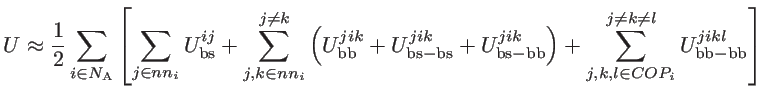(2.18)

where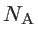,, and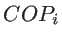are the number of atoms in the system, the number of the nearest neighbors of a specific atom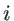, and the coplanar atom groups for atom, respectively. As shown in Fig. 2.5,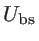,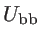,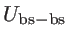,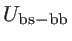, and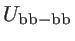are the bond stretching, bond bending, cross bond stretching, cross bond bending stretching, and coplanar bond bending interactions, respectively [45,39]. The terms,, andare an addition to the usual Keating valence force filed (KVFF) model , which can only capture the silicon phononic bandstructure in a limited part of the Brillouin zone. As indicated in Ref.  the introduction of these additional terms provides a more accurate description of the entire Brillouin zone.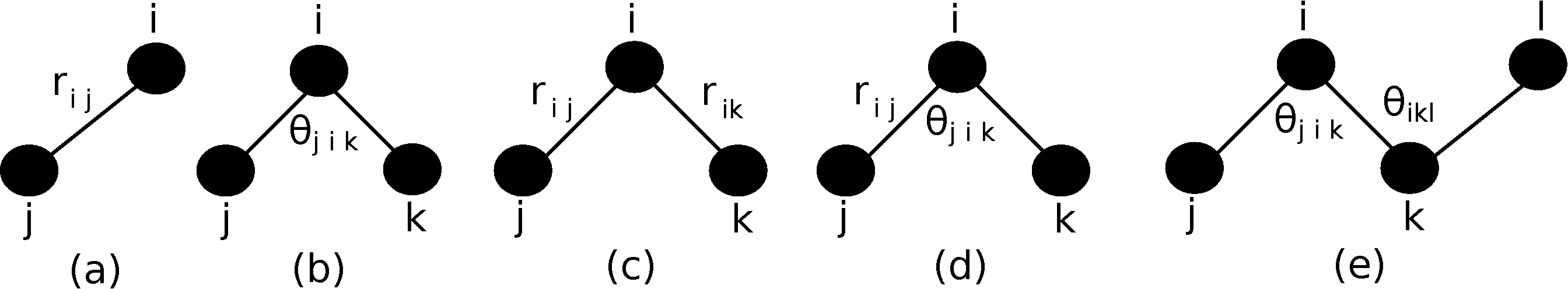These short-range interactions depends on the atomic positions by :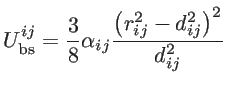(2.19)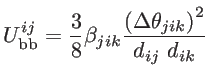(2.20)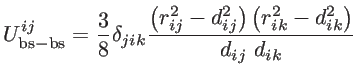(2.21)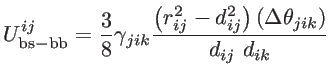(2.22)(2.23)

where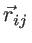and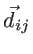are the non-equilibrium and equilibrium bond vectors from atomto atom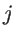, respectively. The angle deviation of bonds betweenand, andand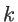is defined by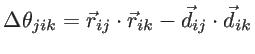. The fitting parameters of silicon,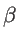,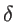,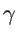, and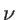are presented in Table 2.2 for both KVFF and MVFF models.Model KVFF  48.5 13.8 0 0 0 MVFF  49.4 4.79 5.2 0.0 6.99

The total potential energy is zero when all the atoms are located in their equilibrium position. Under the harmonic approximation, the motion of atoms can be described by a dynamic matrix as: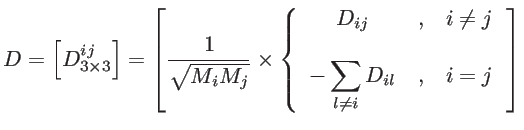(2.24)

where dynamic matrix component between atomsandis given by :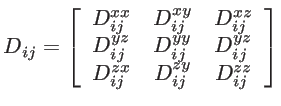(2.25)

and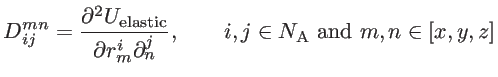(2.26)

is the second derivative of the potential energy with respect to the displacement of atomalong the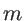-axis and atomalong the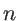-axis.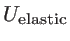is the potential associated with the motion of only two atomsand, whereas the other atoms are considered frozen (unlike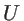, which is the potential when all atoms are allowed to move out of their equilibrium position). To compute: 1) We start withfrom Eq. 2.18. 2) We fix the positions of all atoms except atomsand. 3) We compute the inter-atomic potential due to all bond deformations that result from interaction between both of these two atoms, and sum them up to obtain. All other inter-atomic potential terms that result from interactions due to atomalone, or atomalone, are not considered, since all double derivatives taken with respect to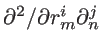, give zero. After setting up the dynamic matrix, the eigenvalue problem can be set up according to Eq. 2.12, the solution of which is the phononic dispersion. Figure 2.6 compares the bulk silicon dispersions calculated using the KVFF and MVFF models with experimental data taken from Ref. . The KVFF fails in some part of the Brillouin zone, whereas the MVFF with three additional terms provides a more accurate description of the entire Brillouin zone.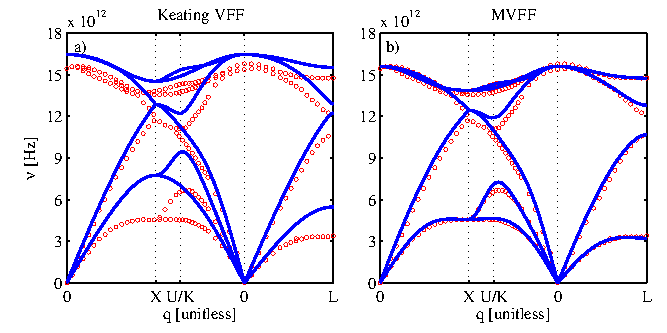Next: 2.3 Electron Transport Up: 2.2 Silicon Previous: 2.2.1 Electronic Structure   Contents
H. Karamitaheri: Thermal and Thermoelectric Properties of Nanostructures